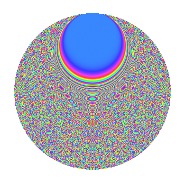# Properties

 Label 1089.2.uLevel 1089 Weight 2 Character orbit u Rep. character $$\chi_{1089}(34,\cdot)$$ Character field $$\Q(\zeta_{33})$$ Dimension 2600 Sturm bound 264

# Related objects

## Defining parameters

 Level: $$N$$ = $$1089 = 3^{2} \cdot 11^{2}$$ Weight: $$k$$ = $$2$$ Character orbit: $$[\chi]$$ = 1089.u (of order $$33$$ and degree $$20$$) Character conductor: $$\operatorname{cond}(\chi)$$ = $$1089$$ Character field: $$\Q(\zeta_{33})$$ Sturm bound: $$264$$

## Dimensions

The following table gives the dimensions of various subspaces of $$M_{2}(1089, [\chi])$$.

Total New Old
Modular forms 2680 2680 0
Cusp forms 2600 2600 0
Eisenstein series 80 80 0

## Trace form

 $$2600q - 11q^{2} - 42q^{3} + 119q^{4} - 9q^{5} - 2q^{6} - 9q^{7} - 32q^{8} - 38q^{9} + O(q^{10})$$ $$2600q - 11q^{2} - 42q^{3} + 119q^{4} - 9q^{5} - 2q^{6} - 9q^{7} - 32q^{8} - 38q^{9} - 44q^{10} - 7q^{11} - 49q^{12} - 9q^{13} - 17q^{14} + 27q^{15} + 119q^{16} - 32q^{17} - 4q^{18} - 48q^{19} - 21q^{20} + 15q^{21} - 11q^{22} - 3q^{23} - 18q^{24} + 113q^{25} - 92q^{26} - 30q^{27} - 60q^{28} - 23q^{29} - 66q^{30} - 9q^{31} - 23q^{32} + 9q^{33} + q^{34} - 44q^{35} - 32q^{36} - 36q^{37} - 57q^{38} + 51q^{39} - 10q^{40} - 23q^{41} - 5q^{42} - 3q^{43} - 64q^{44} + 25q^{45} - 44q^{46} - 21q^{47} + 51q^{48} + 109q^{49} - 5q^{50} - 120q^{51} - 21q^{52} + 76q^{53} - 280q^{54} - 38q^{55} + 21q^{56} - 29q^{57} - 29q^{58} + 15q^{59} - 63q^{60} - 9q^{61} + 16q^{62} + 58q^{63} - 292q^{64} - 29q^{65} + 32q^{66} - 3q^{67} + 7q^{68} + 55q^{69} - 23q^{70} - 100q^{71} - 282q^{72} - 72q^{73} - 65q^{74} - 31q^{75} - 90q^{76} - 135q^{77} - 84q^{78} - 9q^{79} - 544q^{80} - 38q^{81} - 56q^{82} - 11q^{83} - 110q^{84} - 53q^{85} - 55q^{86} + 11q^{87} - 110q^{88} + 58q^{89} - 268q^{90} + 44q^{91} - 91q^{92} + 4q^{93} - 52q^{94} - 135q^{95} + 79q^{96} + 9q^{97} + 96q^{98} + 82q^{99} + O(q^{100})$$

## Decomposition of $$S_{2}^{\mathrm{new}}(1089, [\chi])$$ into newform subspaces

The newforms in this space have not yet been added to the LMFDB.

## Hecke Characteristic Polynomials

There are no characteristic polynomials of Hecke operators in the database